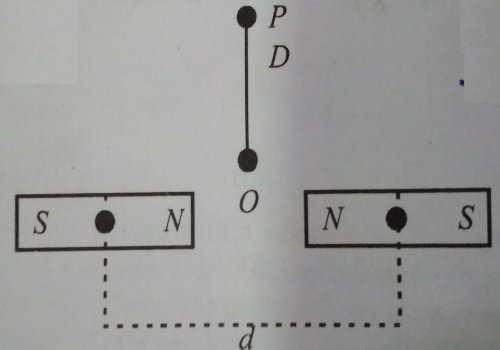Q

# Can someone explain Two identical bar magnets are fixed with their centres at a distance d apart. A stationary charge Q is placed at P in between the gap of the two magnets at a distance D from the centre O as shown in the FigureThe force on the char

Two identical bar magnets are fixed with their centres at a distance d apart. A stationary charge Q is placed at P in between the gap of the two magnets at a distance D from the centre O as shown in the FigureThe force on the charge Q is

• Option 1)

Direct prependicular to the plane of paper

• Option 2)

Zero

• Option 3)

Directed along OP

• Option 4)

Directed along PO

158 Views

Magnetic force If B=0 -

F=0

-

magnetic field apply force on moving charge only. Hence there is no force here on stationary charge

Option 1)

Direct prependicular to the plane of paper

This solution is incorrect

Option 2)

Zero

This solution is correct

Option 3)

Directed along OP

This solution is incorrect

Option 4)

Directed along PO

This solution is incorrect

Exams
Articles
Questions